Next: ADJOINTS AND INVERSES Up: FAMILIAR OPERATORS Previous: Linear interpolation

## Causal integration

Causal integration is defined as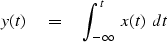(9)
Sampling the time axis gives a matrix equation which we should call causal summation, but we often call it causal integration.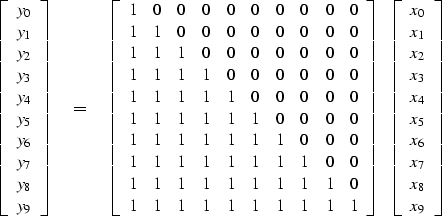(10)
(In some applications the 1 on the diagonal is replaced by 1/2.) Causal integration is the simplest prototype of a recursive operator.

The coding is trickier than operators we considered earlier. Notice when you compute y5 that it is the sum of 6 terms, but that this sum is more quickly computed as y5 = y4 + x5. Thus equation (10) is more efficiently thought of as the recursion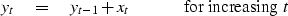(11)
(which may also be regarded as a numerical representation of the differential equation dy/dt=x.)

When it comes time to think about the adjoint, however, it is easier to think of equation (10) than of (11). Let the matrix of equation (10) be called.Transposing to get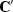and applying it to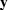gives us something back in the space of,namely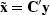.From it we see that the adjoint calculation, if done recursively, needs to be done backwards like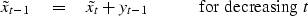(12)
We can sum up by saying that the adjoint of causal integration is anticausal integration.

A subroutine to do these jobs is causint(). The code for anticausal integration is not obvious from the code for integration and the adjoint coding tricks we learned earlier. To understand the adjoint, you need to inspect the detailed form of the expressionand take care to get the ends correct.

# causal integration  (1's on diagonal)
#
temporary real tt( n)
if( adj == 0){                                  tt(1) = xx(1)
do i= 2, n
tt(i) = tt(i-1) + xx(i)
do i= 1, n
yy(i) = yy(i) + tt(i)
}
else {                                          tt(n) = yy(n)
do i= n, 2, -1
tt(i-1) = tt(i) + yy(i-1)
do i= 1, n
xx(i) = xx(i) + tt(i)
}
return; end


 causint Figure 2 in1 is an input pulse. C in1 is its causal integral. C' in1 is the anticausal integral of the pulse. in2 is a separated doublet. Its causal integration is a box and its anticausal integration is the negative. CC in2 is the double causal integral of in2. How can an equilateral triangle be built?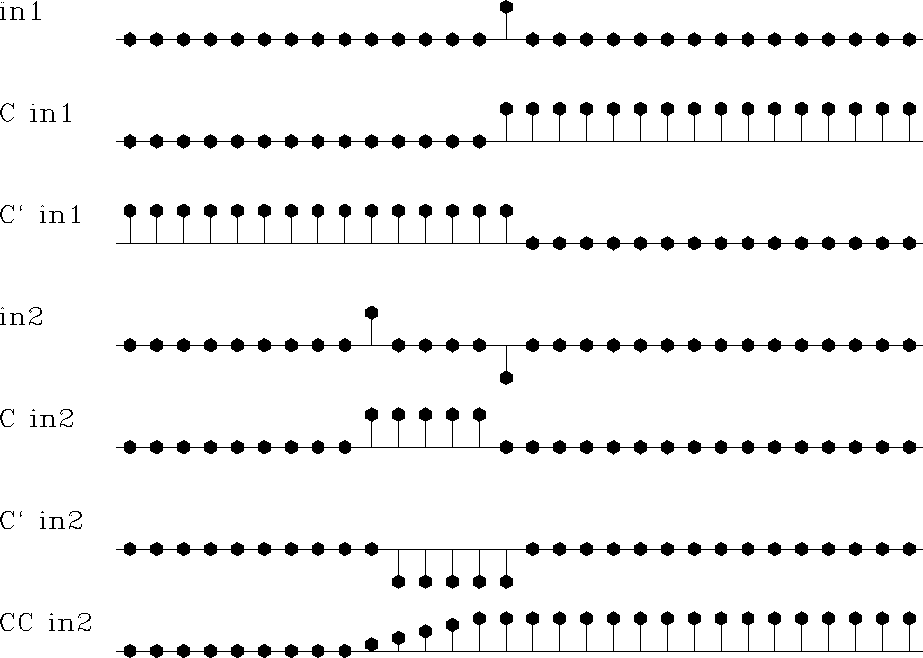Later we will consider equations to march wavefields up towards the earth's surface, a layer at a time, an operator for each layer. Then the adjoint will start from the earth's surface and march down, a layer at a time, into the earth.

## EXERCISES:

1. Modify the calculation in Figure 2 to make a triangle waveform on the bottom row.Next: ADJOINTS AND INVERSES Up: FAMILIAR OPERATORS Previous: Linear interpolation
Stanford Exploration Project
12/26/2000